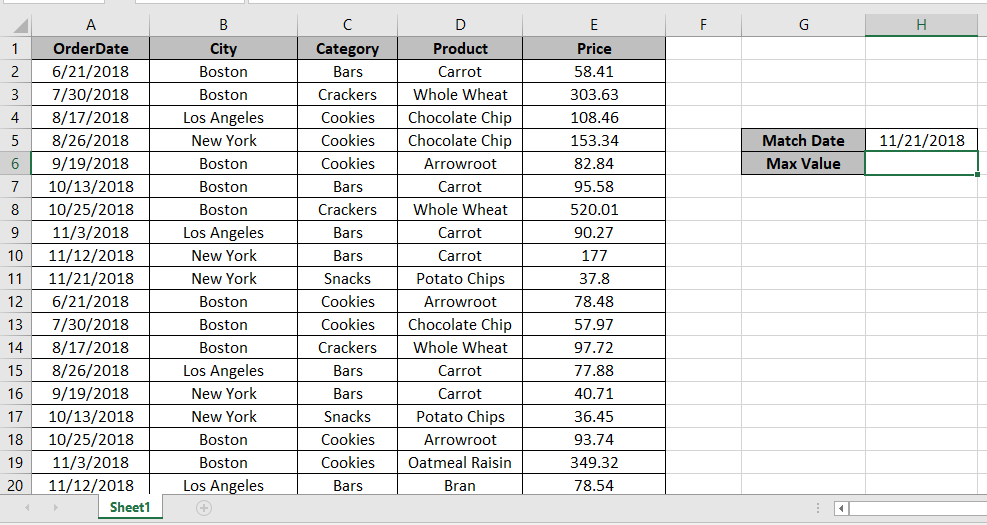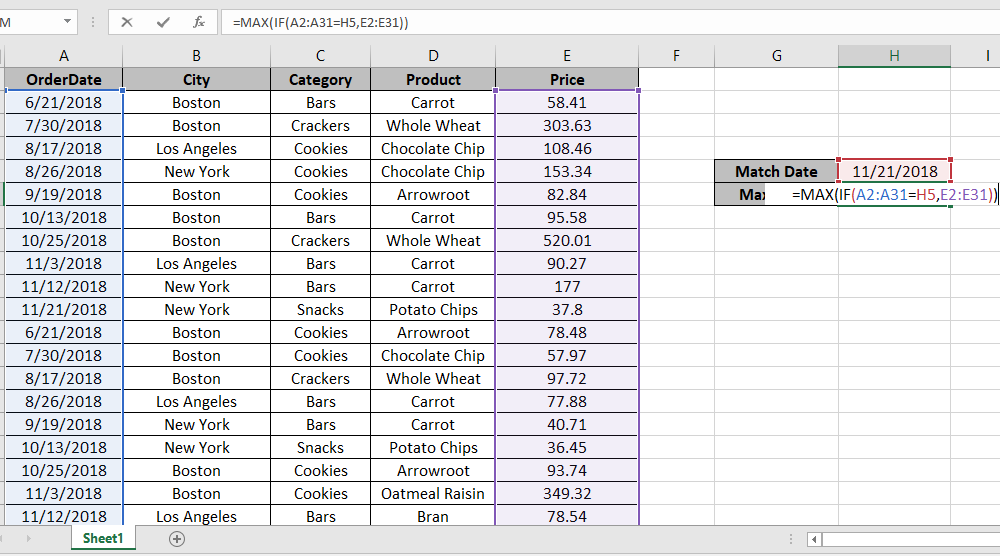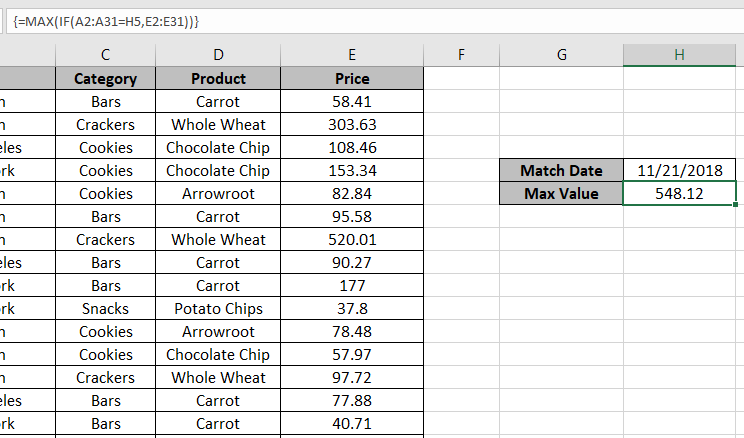# How to Calculate Max if condition match in Excel

In this article, we will learn about how to calculate Maximum value if the condition matches in Excel.

When working with long ranges of data we need to find the max value among the range If given statement stands True.

In simple words, finding out the max value using Excel IF function. IF function returns True or False and Max function looks for the max value corresponding True statement

Syntax to find max if criteria match

{=MAX (IF (Creteria=match, Range_if_true))}

Note: Use Ctrl + Shift + Enter when working with arrays or ranges in Excel. This will generate Curly Braces on the formula by default.

Let’s use this Formula on the data range shown below.Here we have date as match value.

Here the Excel formula to get the max Price from the data set if Date = 11/21/2018.
Use the formula to get the coefficient of variation

{=MAX (IF ( A2:A31 = H5, E2:E31 ))}

Explanation:
Here we matched the range (A2:A31) with the cell value in H5 and returns the Range E2:E31 to find the max from.Press Enter to get the max of the rangeAs you can see we have the MAX value, if criteria match from the range of value

Hope you understood how to get Max value using MAX and IF function in Excel. Explore more articles on Excel function here. Please feel free to state your query or feedback for the above article.

Related Articles:

How to use the MAX function in Excel

How to use MIN function in Microsoft Excel

How to use the LARGE Function in Excel

How to use the SMALL function in Excel

How To Calculate MODE in Excel

How To Calculate Mean in Excel

Popular Articles:

50 Excel Shortcuts to Increase Your Productivity

How to use the VLOOKUP Function in Excel

How to use the COUNTIF function in Excel

How to use the SUMIF Function in Excel

Terms and Conditions of use

The applications/code on this site are distributed as is and without warranties or liability. In no event shall the owner of the copyrights, or the authors of the applications/code be liable for any loss of profit, any problems or any damage resulting from the use or evaluation of the applications/code.